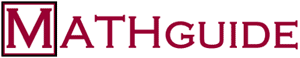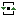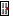A free bulletin board for mathematicseducation. Register to get started. The HELP Center
 You are currently not logged in. You can view the forums, but cannot post messages. | Log In | Register | Search | Help | RefreshWelcome to The HELP Center. If you would like to post a message, you first need to register. It's completely free to register and post messages and only requires the use of an active e-mail account.

Forum Topics Posts Last Post ModeratorsLessonsExisting Math Lessons
Students go here for current online math lessons.Extra Credit
This is the place students go to get special extra credit assignments.Student DialogueMath Talk
This forum is dedicated to a cooperative student exchange of math information.Algebra HelpFunctions
Discover the properties of non-trigonometric functions, like inverses, domain, range, evaluation, ...Order of Operations
Basic computations are handled in this forum.Solving Equations and Inequalities
Solving equations and inequalities is the topic here.Polynomials
Learn about various properties, like zeros, factors, expressions, and graphs.Geometry HelpProofs
Questions and comments related to proofs are handled here.Shapes and Structures
This forum handles questions related to physical shapes and structures, like area, perimeter, volume, ...Counting and ProbabilityProbability
Learn how to calculate the probabilities of various events.Counting
This section addresses counting techniques where the fundamental counting principle, factorials, combinations, and permutations are required.TrigonometryTrigonometry
This section addresses trigonometry: functions, formulas, relationships, identities, the unit circle, ...CalculusCalculus
This forum addresses calculus problems, like limits, derivatives, integrals, related rates, ...Site NewsGeneral Questions and Comments